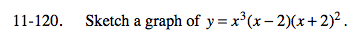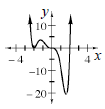### Home > A2C > Chapter Ch11 > Lesson 11.2.6 > Problem11-120

11-120.

Sketch a graph of y = x3(x − 2)(x + 2)2. Homework Help ✎This equation gives you all of the roots of the graph.

The graph touches the x-axis at (−2, 0) and turns around. The graph passes through (0, 0) like a cubic. The graph passes through (2, 0) normally.